# Compare Markov Chain Mixing Times

This example compares the estimated mixing times of several Markov chains with different structures. Convergence theorems typically require ergodic unichains. Therefore, before comparing mixing time estimates, this example ensures that the Markov chains are ergodic unichains.

### Fast-Mixing Chain

Create a 23-state Markov chain from a random transition matrix containing 250 infeasible transitions of 529 total transitions. An infeasible transition is a transition whose probability of occurring is zero. Plot a digraph of the Markov chain and identify classes by using node colors and markers.

```rng(1); % For reproducibility numStates = 23; Zeros1 = 250; mc1 = mcmix(numStates,'Zeros',Zeros1); figure; graphplot(mc1,'ColorNodes',true);```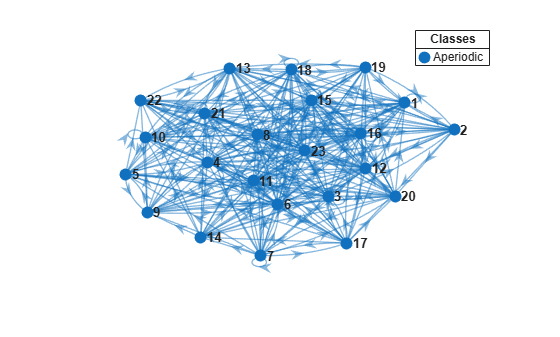`mc1` represents a unichain because it is a single, recurrent, aperiodic class.

Determine whether the Markov chain is ergodic.

`tf1 = isergodic(mc1)`
```tf1 = logical 1 ```

`tf1 = 1` indicates that `mc1` represents an ergodic unichain.

Plot the eigenvalues of the Markov chain on the complex plane.

```figure; eigplot(mc1);```The pink disc in the plot shows the spectral gap (the difference between the two largest eigenvalue moduli). The spectral gap determines the mixing time of the Markov chain. Large gaps indicate faster mixing, whereas thin gaps indicate slower mixing. In this case, the gap is large, indicating a fast-mixing chain.

Estimate the mixing time of the chain.

`[~,tMix1] = asymptotics(mc1)`
```tMix1 = 0.8357 ```

On average, it takes `0.8357` steps for the total variation distance to decay by a factor of ${e}^{1}$.

The expected first hitting time for a target state is another way to view the mixing rate of a Markov chain. The hitting time computation does not require an ergodic Markov chain.

Plot a digraph of the Markov chain with node colors representing the expected first hitting times for regime 1.

`hittime(mc1,1,'Graph',true);`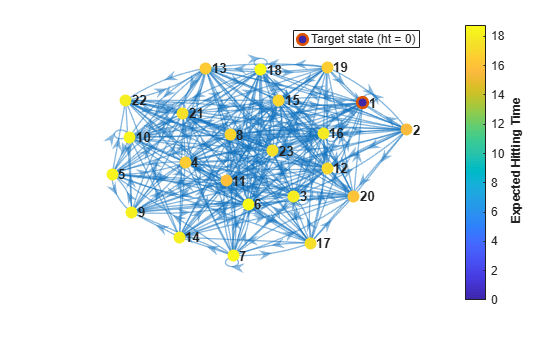The expected first hitting time for regime 1 beginning from regime 2 is approximately 16 time steps.

### Slow-Mixing Chain

Create another 23-state Markov chain from a random transition matrix containing 475 infeasible transitions. With fewer feasible transitions, this chain should take longer to mix. Plot a digraph of the Markov chain and identify classes by using node colors and markers.

```Zeros2 = 475; mc2 = mcmix(numStates,'Zeros',Zeros2); figure; graphplot(mc2,'ColorNodes',true);```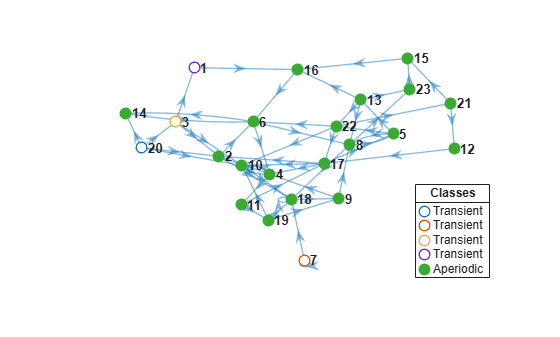`mc2` represents a unichain because it has a single, recurrent, aperiodic class and several transient classes.

Determine whether the Markov chain is ergodic.

`tf2 = isergodic(mc2)`
```tf2 = logical 0 ```

`tf2 = 0` indicates that `mc2` is not ergodic.

Extract the recurrent subchain from `mc2`. Determine whether the subchain is ergodic.

```[bins,~,ClassRecurrence] = classify(mc2); recurrentClass = find(ClassRecurrence,1); recurrentState = find((bins == recurrentClass),1); sc2 = subchain(mc2,recurrentState); tf2 = isergodic(sc2)```
```tf2 = logical 1 ```

`sc2` represents an ergodic unichain.

Plot the eigenvalues of the subchain on the complex plane.

```figure; eigplot(sc2);```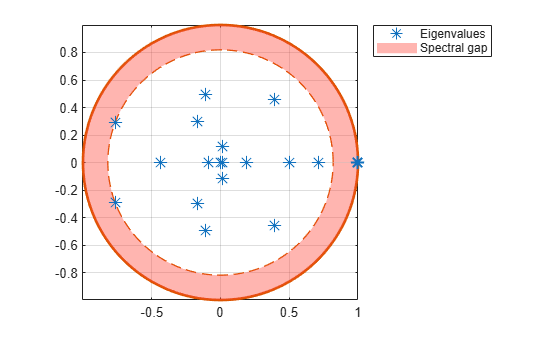The spectral gap in the subchain is much thinner than the gap in `mc1`, which indicates that the subchain mixes more slowly.

Estimate the mixing time of the subchain.

`[~,tMix2] = asymptotics(sc2)`
```tMix2 = 5.0201 ```

On average, it takes `5.0201` steps for the total variation distance to decay by a factor of ${e}^{1}$.

Plot a digraph of the Markov chain with node colors representing the expected first hitting times for the first regime in the recurrent subclass.

`sc2.StateNames(1)`
```ans = "2" ```
`hittime(sc2,1,'Graph',true);`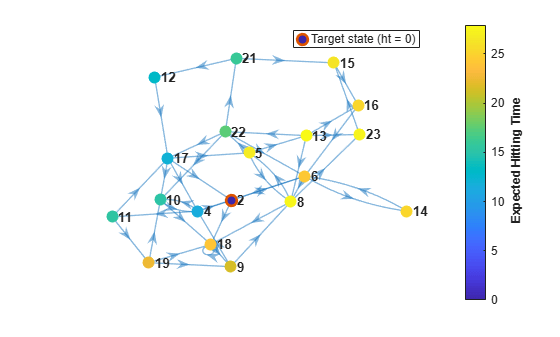The expected first hitting time for regime 2 beginning from regime 8 is about 30 time steps.

### Dumbbell Chain Mixing Time

Create a "dumbbell" Markov chain containing 10 states in each "weight" and three states in the "bar."

• Specify random transition probabilities between states within each weight.

• If the Markov chain reaches the state in a weight that is closest to the bar, then specify a high probability of transitioning to the bar.

• Specify uniform transitions between states in the bar.

```w = 10; % Dumbbell weights DBar = [0 1 0; 1 0 1; 0 1 0]; % Dumbbell bar DB = blkdiag(rand(w),DBar,rand(w)); % Transition matrix % Connect dumbbell weights and bar DB(w,w+1) = 1; DB(w+1,w) = 1; DB(w+3,w+4) = 1; DB(w+4,w+3) = 1; mc3 = dtmc(DB);```

Plot a directed graph of the dumbbell chain and identify classes by using node colors and markers. Suppress node labels.

```figure; h = graphplot(mc3,'ColorNodes',true); h.NodeLabel = {};```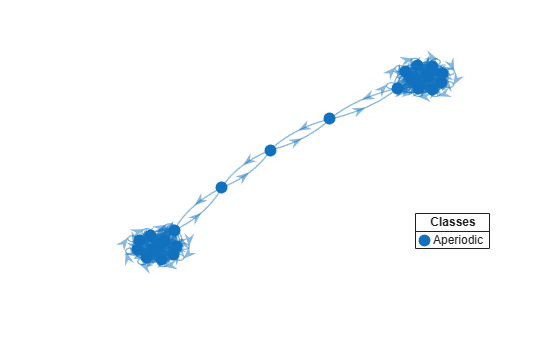`mc3` represents a unichain because it has a single, recurrent, aperiodic class.

Determine whether the Markov chain is ergodic.

`tf3 = isergodic(mc3)`
```tf3 = logical 1 ```

`tf3 = 1` indicates that `mc3` is ergodic.

Plot the eigenvalues of the dumbbell on the complex plane.

```figure; eigplot(mc3);```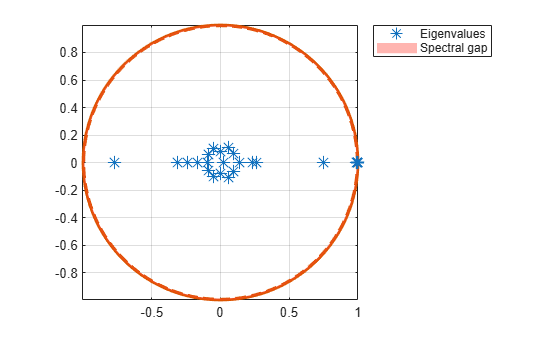The spectral gap in the subchain is very thin, which indicates that the dumbbell chain mixes very slowly.

Estimate the mixing time of the dumbbell chain.

`[~,tMix3] = asymptotics(mc3)`
```tMix3 = 90.4334 ```

On average, it takes `90.4334` steps for the total variation distance to decay by a factor of ${e}^{1}$.

Plot a digraph of the Markov chain with node colors representing the expected first hitting times for regime 1.

`hittime(mc3,1,'Graph',true);`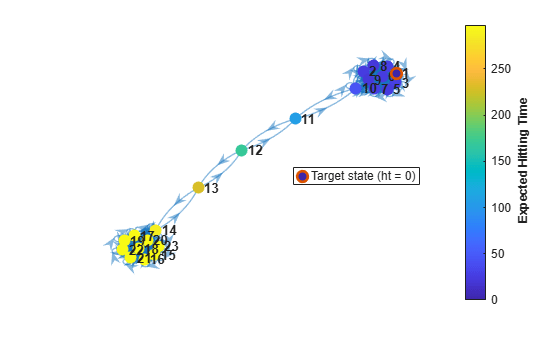The expected first hitting time for regime 1 beginning from regime 15 is about 300 time steps.